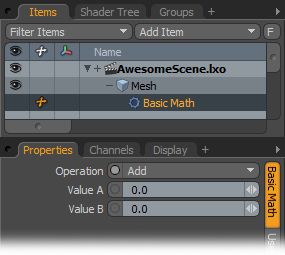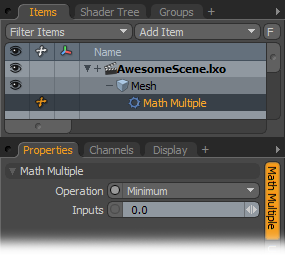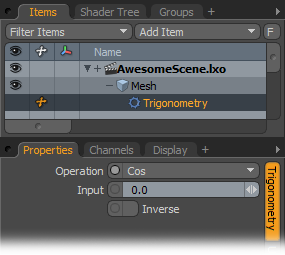# Basic MathWith this Channel Modifier Modo performs basic math operations and passes the result to a channel that can then be read by other Channel Modifiers or linked to a compatible numeric channel on any item. The inputs can be constant or animated values on the Value A or Value B channels of the modifier itself, or you can link values from other channels. The channel values for the inputs are A and B. The output value is C.

Operations: You can select from these basic math operations:
Add- Value A + Value B = Output C
Subtract- Value A - Value B = Output C
Multiply- Value A * Value B = Output C
Divide- Value A / Value B = Output C
Modulo- The remainder of Value A / Value B = Output C
Average- The average of Value A and Value B = Output C
Minimum- The lesser of Value A and Value B = Output C
Maximum- The greater of Value A and Value B = Output C

Value A/Value B: You can link the input of other channels into this modifier to calculate the output value and link the output channel to the target channel.

# Math MultipleYou can define multiple inputs for the math operation and then pass the result to the result (C) output channel.

Operations: You can select from these basic math operations:

Add- Value A + Value B = Output C

Subtract- Value A - Value B = Output C

Multiply- Value A * Value B = Output C

Divide- Value A / Value B = Output C

Average- The average of Value A and Value B = Output C

Minimum- The lesser of Value A and Value B = Output C

Maximum- The greater of Value A and Value B = Output C

Inputs: This is the input value for the numeric calculation. Typically, you link this to another channel.

# Math

Modo has additional math operators available for calculating other values. As with the other math modifiers, you can link channels to the Input channel. Modo feeds the resulting values through the Output channel.

Operations: You can select from these additional math operations:

Absolute- Calculates the absolute value for a number regardless of its sign (for example, 3=3 and -3=3).

Ceiling- Calculates a maximum integer (whole number) value.

Constant- Generates common, constant numerical values such as Pi, Eulers number, and the Golden Ratio.

Exp- Calculates the exponent of the input value based on the indicated Exponent value (which can also be used as an input).

Floor- Calculates a minimum integer (whole number) value.

Log- Calculates the natural logarithmic value.

Log10- Calculates the common logarithmic value.

Power- Calculates the exponential value for a number based on the input Power.

Square Root- Calculates the square root of the input value.

# TrigonometryYou can apply trigonometric operations to a channel. Trigonometric operations relate the angles of a triangle to the lengths of the sides of the triangle.

Operation:

Cosine - Indicates the ratio of the adjacent side to the hypotenuse of a right-angled triangle.

Sine - Indicates the ratio of the length of the side opposite the given angle to the length of the hypotenuse of a right-angled triangle.

Tangent - Indicates the ratio of the side opposite the angle to the adjacent side of a right-angled triangle.

Input: This is the input value for the numeric calculation. Typically, you link another channel to provide this value.

Inverse: If you select this, Modo inverts the output value.